Finite Geometry Notes   | Home | Site Map | Author |

# Finite Relativity

From the journal of Steven H. Cullinane:

Today, Feb. 20, 2004, is the 18th birthday of my note "The Relativity Problem in Finite Geometry" shown below.

That note begins with a quotation from Weyl:

"This is the relativity problem: to fix objectively a class of equivalent coordinatizations and to ascertain the group of transformations S mediating between them."
-- Hermann Weyl, The Classical Groups, Princeton University Press, 1946, p. 16

Here is another quotation from Weyl, on the profound branch of mathematics known as Galois theory, which he says

"... is nothing else but the relativity theory for the set Sigma, a set which, by its discrete and finite character, is conceptually so much simpler than the infinite set of points in space or space-time dealt with by ordinary relativity theory."
-- Weyl, Symmetry, Princeton University Press, 1952, p. 138

This second quotation applies equally well to the much less profound, but more accessible, part of mathematics described in Diamond Theory and in my note below.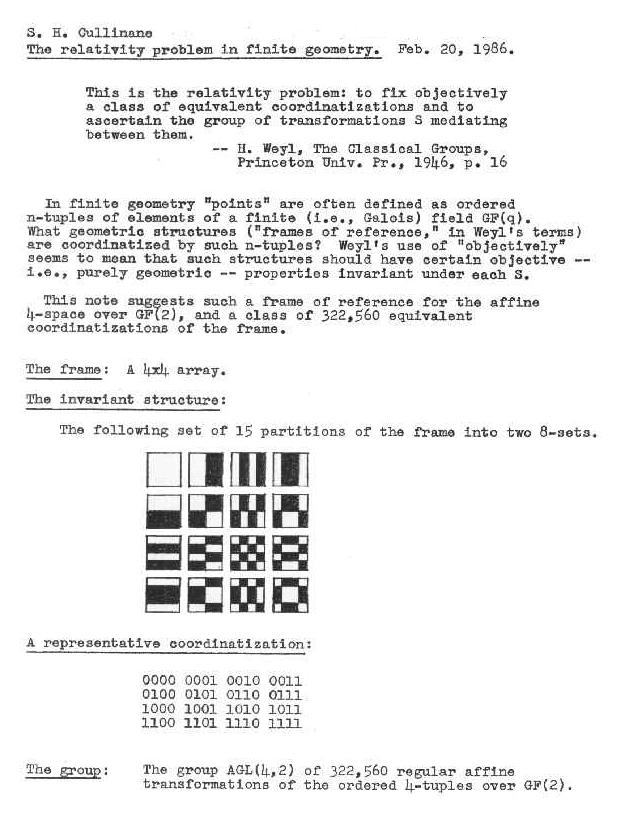#### On the order of AGL(4,2):

See Groups and Symmetry, by Phill Schultz.
See especially Part 19, Linear Groups Over Other Fields.

As Schultz demonstrates, the order of AGL(4,2), the affine group
in the four-dimensional space over the two-element field, is

(24)(24 - 1)(24 - 21)(24 - 22)(24 - 23) =
(16)(16 - 1)(16 - 2)(16 - 4)(16 - 8) =
(16)(15)(14)(12)(8) = 322,560.

This group can be generated by arbitrarily mixing
permutations of rows and columns in the 4x4 array
with permutations of the array's four quadrants.
For a proof, see Binary Coordinate Systems.

Related material:

Invariants

"What modern painters are trying to do,
if they only knew it, is paint invariants."

-- James J. Gibson in Leonardo
(Vol. 11, pp. 227-235.
Pergamon Press Ltd., 1978)

An example of invariant structure: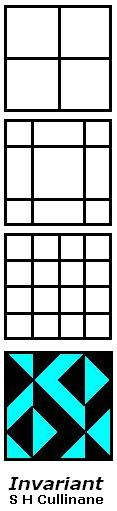The three line diagrams above result from the three partitions, into pairs of 2-element sets, of the 4-element set from which the entries of the bottom colored figure are drawn.  Taken as a set, these three line diagrams describe the structure of the bottom colored figure.  After coordinatizing the figure in a suitable manner, we find that the line diagrams are invariant under the group of 16 binary translations acting on the colored figure.

For another sort of invariance of the colored figure, try applying a symmetry of the square to each of the set of four diagonally-divided squares from which the figure's entries are drawn, and observe the induced effect on the figure itself.

A more remarkable invariance -- that of symmetry itself -- is observed if we arbitrarily and repeatedly permute rows and/or columns and/or 2x2 quadrants of the colored figure above. Each resulting figure has some ordinary or color-interchange symmetry. The cause of this symmetry-invariance in the colored patterns is the symmetry-invariance of the line diagrams under a group of 322,560 binary affine transformations.

For more details on the above invariant structures, see Geometry of the 4x4 Square, the Diamond 16 Puzzle, and Diamond Theory.

Also perhaps relevant:

 Einstein wanted to know what was invariant (the same) for all observers. Some felt that his relativity theory should be called "theory of invariants." (See below.)

For another perspective, see Block Designs in Art and Mathematics.

Some further remarks

of Feb. 20, 2007,
the 21st birthday
of the above note:

Weyl's set Sigma is a finite set of complex numbers.   Some other sets with "discrete and finite character" are those of 4, 8, 16, or 64 points, arranged in squares and cubes.  For illustrations, see Finite Geometry of the Square and Cube.  What Weyl calls "the relativity problem" for these sets involves fixing "objectively" a class of equivalent coordinatizations.  For what Weyl's "objectively" means, see the article "Symmetry and Symmetry  Breaking," by Katherine Brading and Elena Castellani, in the Stanford Encyclopedia of Philosophy:

"The old and natural idea that what is objective should not depend upon the particular perspective under which it is taken into consideration is thus reformulated in the following group-theoretical terms: what is objective is what is invariant with respect to the transformation group of reference frames, or, quoting Hermann Weyl (1952, p. 132), 'objectivity means invariance with respect to the group of automorphisms [of space-time].'

22. The significance of the notion of invariance and its group-theoretic treatment for the issue of objectivity is explored in Born (1953), for example. For more recent discussions see Kosso (2003) and Earman (2002, Sections 6 and 7).

References:

Born, M., 1953, "Physical Reality," Philosophical Quarterly, 3, 139-149. Reprinted in E. Castellani (ed.), Interpreting Bodies: Classical and Quantum Objects in Modern Physics, Princeton, NJ: Princeton University Press, 1998, pp. 155-167.

Earman, J., 2002, "Laws, Symmetry, and Symmetry Breaking; Invariance, Conservation Principles, and Objectivity,' PSA 2002, Proceedings of the Biennial Meeting of the Philosophy of Science Association 2002, forthcoming [Abstract/Preprint available online]

Kosso, P., 2003, "Symmetry, objectivity, and design," in K. Brading and E. Castellani (eds.), Symmetries in Physics: Philosophical Reflections, Cambridge: Cambridge University Press, pp. 410-421.

Weyl, H., 1952, Symmetry, Princeton, NJ: Princeton University Press.

On relativity theory as "theory of invariants"--

See Archives Henri Poincaré (research unit UMR 7117, at Université Nancy 2, of the CNRS)--

"Minkowski, Mathematicians, and the Mathematical Theory of Relativity," by Scott Walter, in The Expanding Worlds of General Relativity (Einstein Studies, volume 7), H. Goenner, J. Renn, J. Ritter and T. Sauer, editors, Boston/Basel: Birkhäuser, 1999, pp. 45-86--

"Developing his ideas before Göttingen mathematicians in April 1909, Klein pointed out that the new theory based on the Lorentz group (which he preferred to call 'Invariantentheorie') could have come from pure mathematics (1910: 19). He felt that the new theory was anticipated by the ideas on geometry and groups that he had introduced in 1872, otherwise known as the Erlangen program (see Gray 1989: 229)."

References:

Gray, Jeremy J. (1989). Ideas of Space. 2d ed. Oxford: Oxford University Press.

Klein, Felix. (1910). "Über die geometrischen Grundlagen der Lorentzgruppe." Jahresbericht der deutschen Mathematiker-Vereinigung 19: 281-300. [Reprinted: Physikalische Zeitschrift 12 (1911): 17-27].

Update of Feb. 20, 2008:

For some remarks on the relativity problem in the simpler case of a 2x2x2 cube rather than a 4x4 square, see Knight Moves: Geometry of the Eightfold Cube.

Update of May 16, 2008:

From some 1949 remarks of Weyl:

"The relativity problem is one of central significance throughout geometry and algebra and has been recognized as such by the mathematicians at an early time."
-- Hermann Weyl, "Relativity Theory as a Stimulus in Mathematical Research," Proceedings of the American Philosophical Society, Vol. 93, No. 7, Theory of Relativity in Contemporary Science: Papers Read at the Celebration of the Seventieth Birthday of Professor Albert Einstein in Princeton, March 19, 1949 (Dec. 30, 1949), pp. 535-541

Update of February 20, 2010:

Weyl on what he calls the relativity problem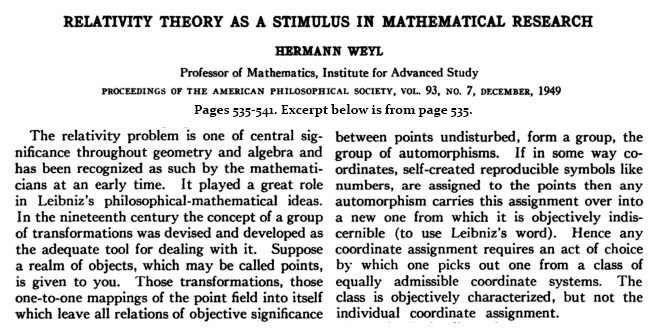(Image added on Sept. 20, 2011)

"The relativity problem is one of central significance throughout geometry and algebra and has been recognized as such by the mathematicians at an early time."

– Hermann Weyl, 1949, "Relativity Theory as a Stimulus in Mathematical Research"

"This is the relativity problem: to fix objectively a class of equivalent coordinatizations and to ascertain the group of transformations S mediating between them."

– Hermann Weyl, 1946, The Classical Groups, Princeton University Press, p. 16

Twenty-four years ago a note of Feb. 20, 1986, supplied an example of such coordinatizations in finite geometry. In that note, the group of mediating transformations acted directly on  coordinates within a 4×4 array. When the 4×4 array is embedded in a 4×6 array, a larger and more interesting group, M24 (containing the original group), acts on the larger array.  There is no obvious solution to Weyl's relativity problem for M24.  That is, there is no obvious way* to apply exactly 24 distinct transformable coordinate-sets (or symbol-strings) to the 24 array elements in such a way that the natural group of mediating transformations of the 24 symbol-strings is M24.

There is, however, an assignment of symbol-strings that yields a family of sets with automorphism group M24.

R.D. Carmichael in 1931 on his construction of the Steiner system S(5,8,24)–

"The linear fractional group modulo 23 of order 24•23•11 is often represented as a doubly transitive group of degree 24 on the symbols ∞, 0, 1, 2,…, 22. This transitive group contains a subgroup of order 8 each element of which transforms into itself the set ∞, 0, 1, 3, 12, 15, 21, 22 of eight elements, while the whole group transforms this set into 3•23•11 sets of eight each. This configuration of octuples has the remarkable property that any given set of five of the 24 symbols occurs in one and just one of these octuples. The largest permutation group Γ on the 24 symbols, each element of which leaves this configuration invariant, is a five-fold transitive group of degree 24 and order 24•23•22•21•20•48. This is the Mathieu group of degree 24."

– R. D. Carmichael, 1931, "Tactical Configurations of Rank Two," in American Journal of Mathematics, Vol. 53, No. 1 (Jan., 1931), pp. 217-240

Footnote of  Sept. 20, 2011 to the update of  Feb. 20, 2010--

* R.T. Curtis has, it seems, a non-obvious way that involves strings of seven symbols.  His abstract for a 1990 paper says that in his construction "The generators of M24 are defined... as permutations of twenty-four 7-cycles in the action of PSL2(7) on seven letters...."

See "Geometric Interpretations of the ‘Natural’ Generators of the Mathieu groups," by R.T. Curtis,  Mathematical Proceedings of the Cambridge Philosophical Society (1990), Vol. 107, Issue 01, pp. 19-26. (Rec. Jan. 3, 1989, revised Feb. 3, 1989.) This paper was published online on Oct. 24, 2008.

Some related articles by Curtis:

R.T. Curtis, "Natural Constructions of the Mathieu groups," Math. Proc. Cambridge Philos. Soc.  (1989), Vol. 106, pp. 423-429 (Rec. Nov. 16, 1988, revised Jan. 3, 1989.)

R.T. Curtis. "Symmetric Presentations I: Introduction, with Particular Reference to the Mathieu groups M 12  and M 24" In Proceedings of 1990 LMS Durham Conference 'Groups, Combinatorics and Geometry'  (eds. M. W. Liebeck and J. Saxl),  London Math. Soc. Lecture Note Series 165, Cambridge University Press, 1992, pp. 380–396

R.T. Curtis, "A Survey of Symmetric Generation of Sporadic Simple Groups," in The Atlas of Finite Groups: Ten Years On, (eds. R.T. Curtis and R.A. Wilson), London Math. Soc. Lecture Note Series 249, Cambridge University Press, 1998, pp. 39–57

Update of February 20, 2012:

### Coxeter and the Relativity Problem

In the Beginning…

"As is well known, the Aleph is the first letter of the Hebrew alphabet."
– Borges, "The Aleph" (1945)

From some 1949 remarks of Weyl

"The relativity problem is one of central significance throughout geometry and algebra and has been recognized as such by the mathematicians at an early time."

Hermann Weyl, "Relativity Theory as a Stimulus in Mathematical Research," Proceedings of the American Philosophical Society , Vol. 93, No. 7, Theory of Relativity in Contemporary Science: Papers Read at the Celebration of the Seventieth Birthday of Professor Albert Einstein in Princeton, March 19, 1949  (Dec. 30, 1949), pp. 535-541

Weyl in 1946—

"This is the relativity problem: to fix objectively a class of equivalent coordinatizations and to ascertain the group of transformations S mediating between them."

– Hermann Weyl, The Classical Groups , Princeton University Press, 1946, p. 16

Coxeter in 1950 described the elements of the Galois field GF(9) as powers of a primitive root and as ordered pairs of the field of residue-classes modulo 3—

"… the successive powers of  the primitive root λ or 10 are

λ = 10,  λ2 = 21,  λ3 = 22,  λ4 = 02,
λ5 = 20,  λ6 = 12,  λ7 = 11,  λ8 = 01.

These are the proper coordinate symbols….

(See Fig. 10, where the points are represented in the Euclidean plane as if the coordinate residue 2 were the ordinary number -1. This representation naturally obscures the collinearity of such points as λ4, λ5, λ7.)"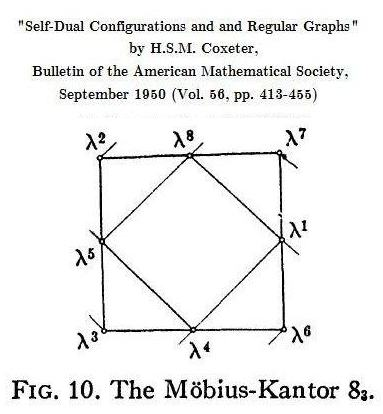Coxeter's Figure 10 yields...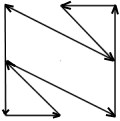The Aleph

The details: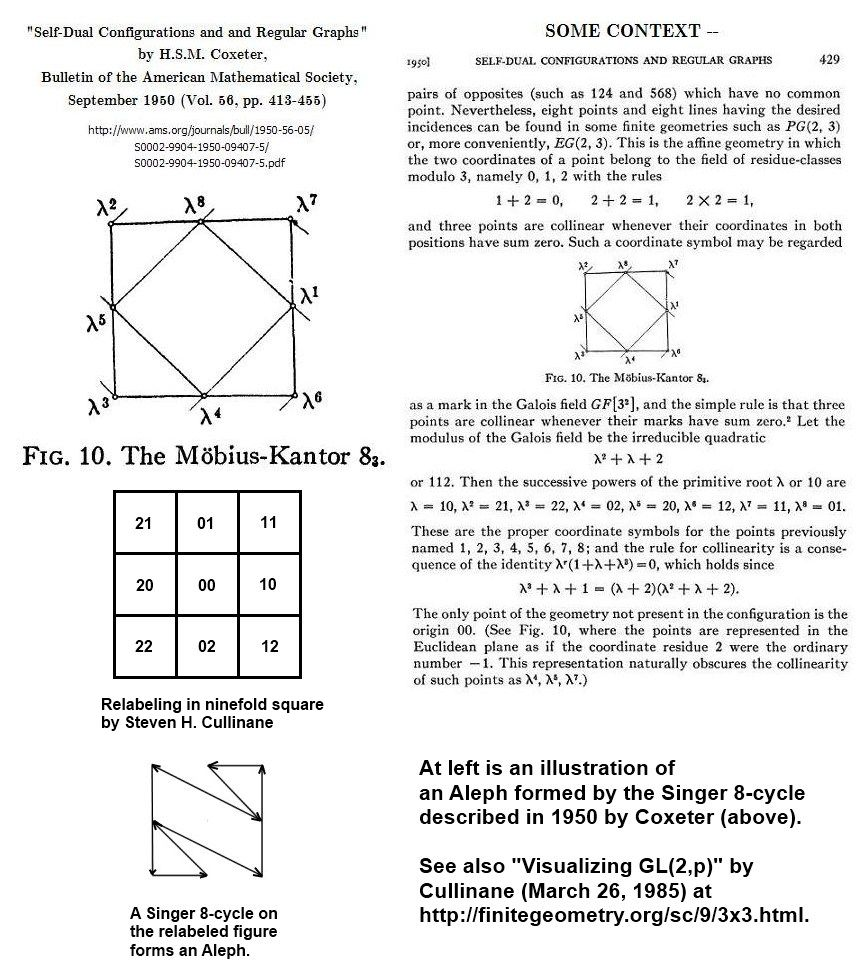Coxeter's phrase "in the Euclidean plane" obscures the noncontinuous nature
of the transformations that are automorphisms of the above linear 2-space over GF(3).